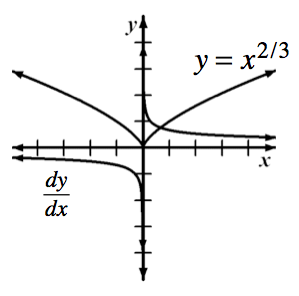### Home > APCALC > Chapter 3 > Lesson 3.4.4 > Problem3-188

3-188.

ANOTHER PROBLEM FOR HANAH

1. Graph $f(x) = x^{2/3}$ on graph paper. Then without a calculator, sketch $y = f ^\prime (x)$ on the same set of axes.2. Describe the graphs of $y = f(x)$ and $y = f ^\prime (x)$ at $x = 0$.

3. Use your graphing calculator to determine the slope of $f(x) = x^{2/3}$ at the vertex.

Though the derivative does not exist, some graphing calculators (inaccurately) say that the derivative is $0$ at a cusp.

4. Calculators are not always accurate. Some graphing calculators incorrectly determine slopes at a vertex, as well as other cusps, because they use the symmetric difference quotient to calculate the slope of a tangent line.

For $f(x) = x^{2/3}$, use $\frac { f ( x + h ) - f ( x - h ) } { 2 h }$, to calculate $f ^\prime (0)$ for $h = 0.1, −0.1,$ and $0.01$. What do you notice? What leads a calculator to give a false derivative of $f(x) = x^{2/3}$ at $x = 0$?